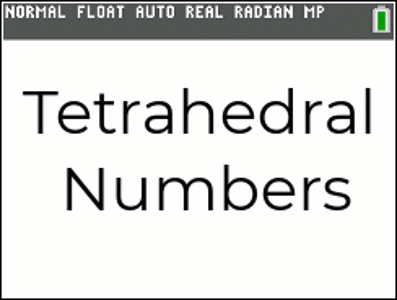•• ##### Device
•TI-84 Plus CE

# QLD (TI-84PlusCE): Part 2 of 3 - Tetrahedral Induction

by Texas Instruments#### Objectives

Part 2 of 3: Induction - students build equations visually, graphically and numerically and then prove their formula to be true for all whole numbers by Mathematical Induction. Additional questions are provided for students to practice the process of Mathematical Induction.

#### Vocabulary

• Induction
• Tetrahedral Numbers
• Sum
• Cubic